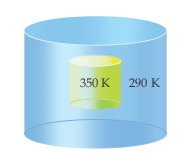# Problem: Imagine a container placed in a tub of water, as depicted in the accompanying diagram.If neither the volume nor the pressure of the system changes during the process, how is the change in internal energy related to the change in enthalpy?

###### FREE Expert Solution

Internal Energy:

$\overline{){\mathbf{∆}}{\mathbf{E}}{\mathbf{=}}{\mathbf{q}}{\mathbf{+}}{\mathbf{w}}}$

Recall that work (w) is given by:

$\overline{){\mathbf{w}}{\mathbf{=}}{\mathbf{-}}{\mathbf{P\Delta V}}}$

• (+): heat, q is gained by the system (i.e. endothermic rxn )

• (–): heat, q is released by the system (i.e. exothermic rxn)

• (+): work is done by the surroundings to the system (compression)

• (–): work is done by the system to the surroundings (expansion)

At constant P → q = ΔH (enthalpy)

At constant V → w = 0

94% (56 ratings)###### Problem Details

Imagine a container placed in a tub of water, as depicted in the accompanying diagram.If neither the volume nor the pressure of the system changes during the process, how is the change in internal energy related to the change in enthalpy?

Frequently Asked Questions

What scientific concept do you need to know in order to solve this problem?

Our tutors have indicated that to solve this problem you will need to apply the Internal Energy concept. You can view video lessons to learn Internal Energy. Or if you need more Internal Energy practice, you can also practice Internal Energy practice problems.